# Exponential Regression Calculator

Or input your data as csv

Sharing helps us build more free tools

Fit a y = ae^bx line of best fit with this free online exponential regression calculator.

Exponential regression is used to find an exponential curve that best fits the input data.

The chart below shows an exponential regression fit on field efficiency vs engine displacement for cars in the mtcars data set.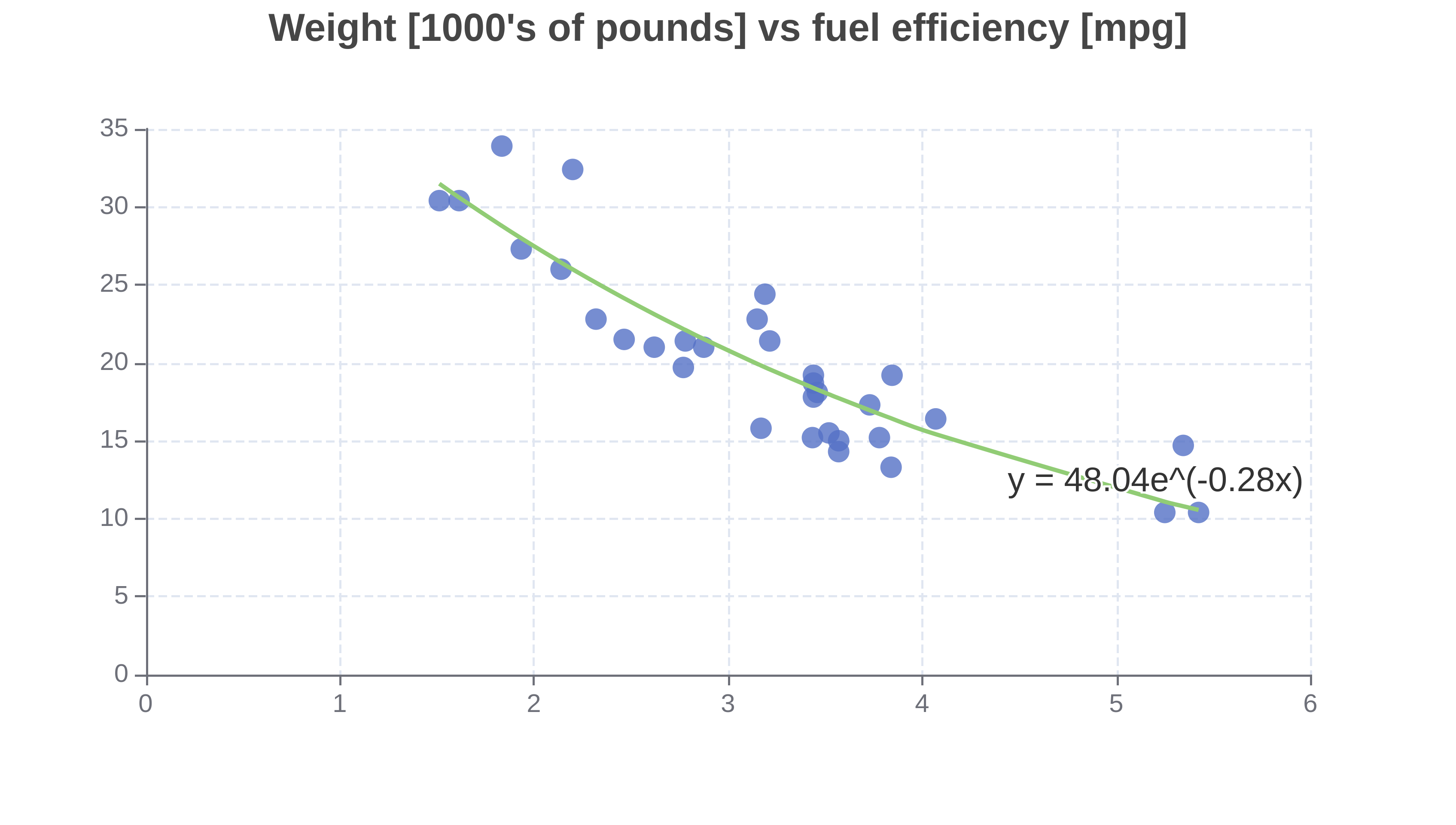Exponential growth curves start by growing slowly and then begin to grow very quickly.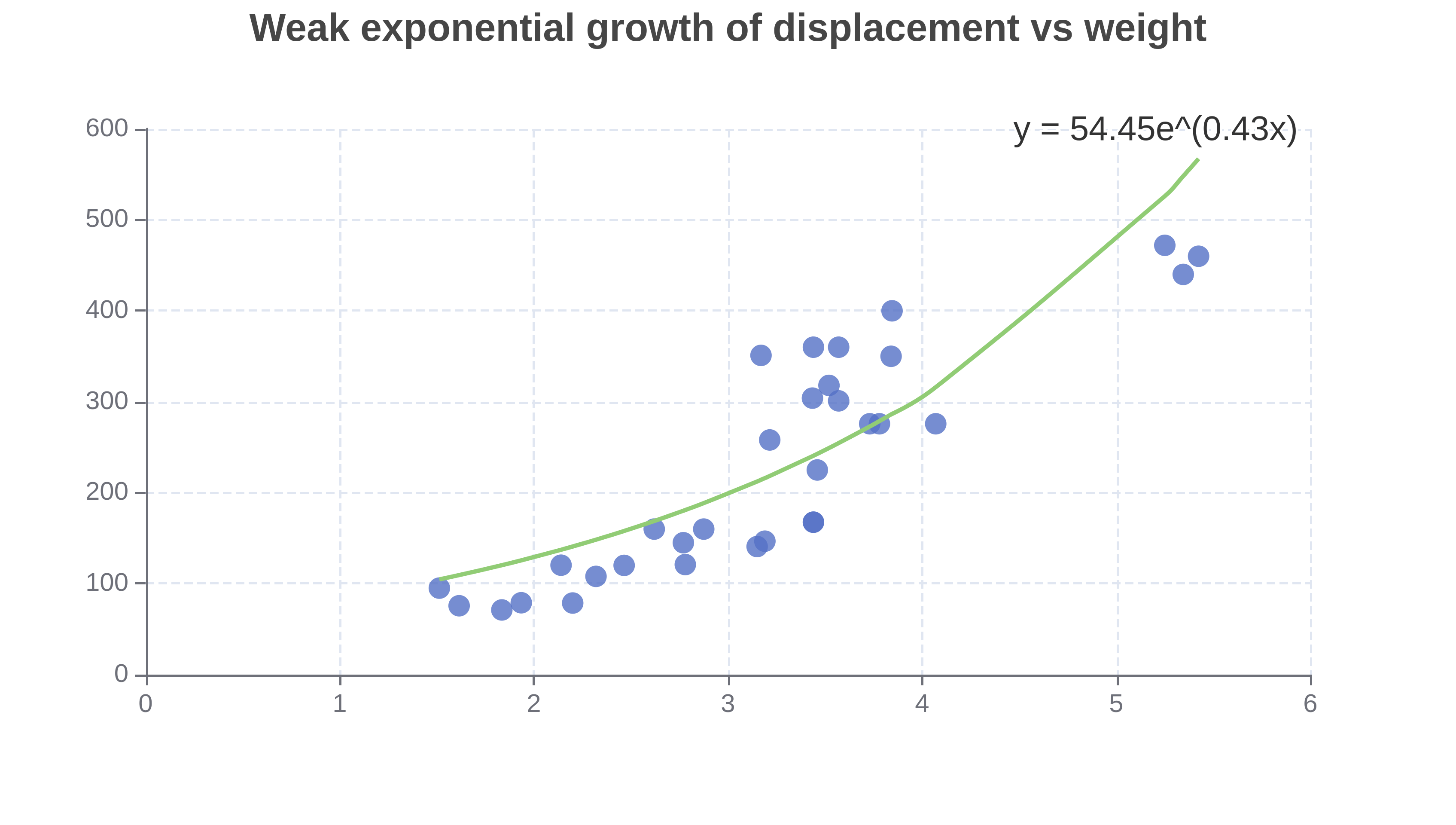Exponential decay curves start by decreasing slowly and then begin to decay very quickly.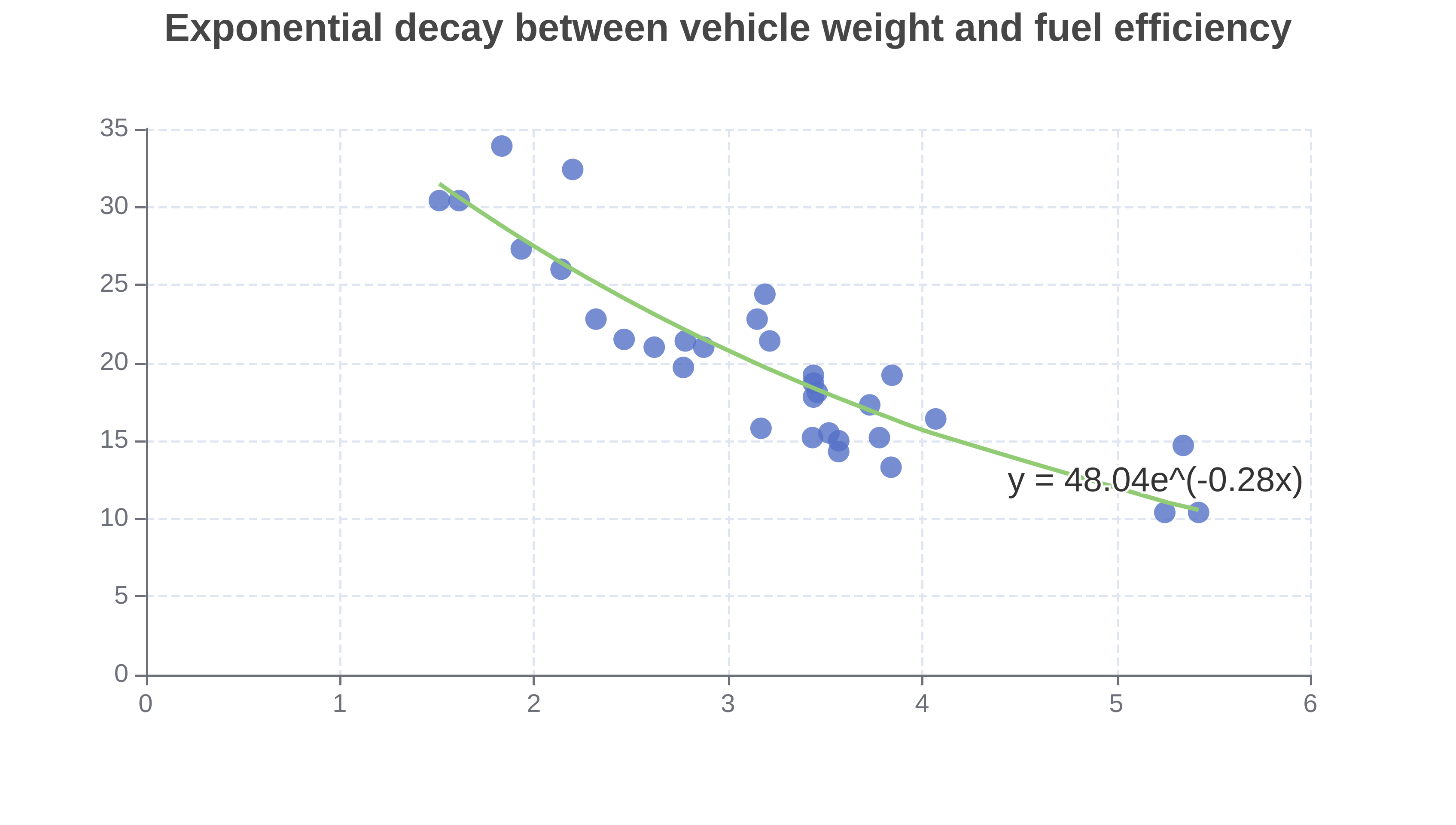## When To Use Exponential Regression

Exponential regression is best used when the input data can be modeled by an exponential function. It has a sharp "hockey stick" shape. The input data might look like an exponential growth or decay curve.

This exponential regression calculator is not always the best tool for the job. Sometimes one of the other related calculators might be more useful.

The linear regression calculator is better suited if your data looks like a straight line.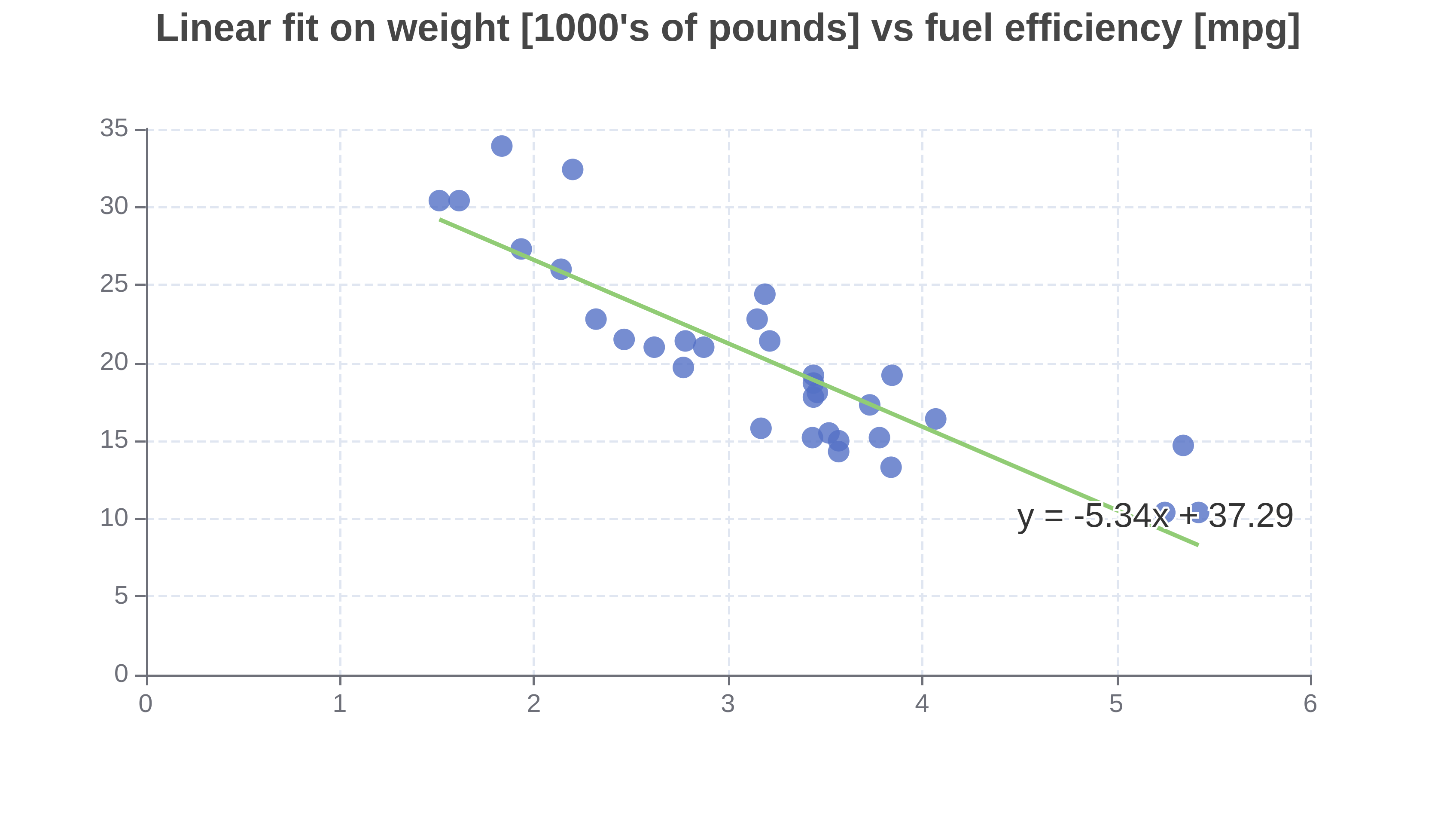Quadratic regression or  polynomial regression are good tools when your data does not look linear but is not changing as fast as an exponential function.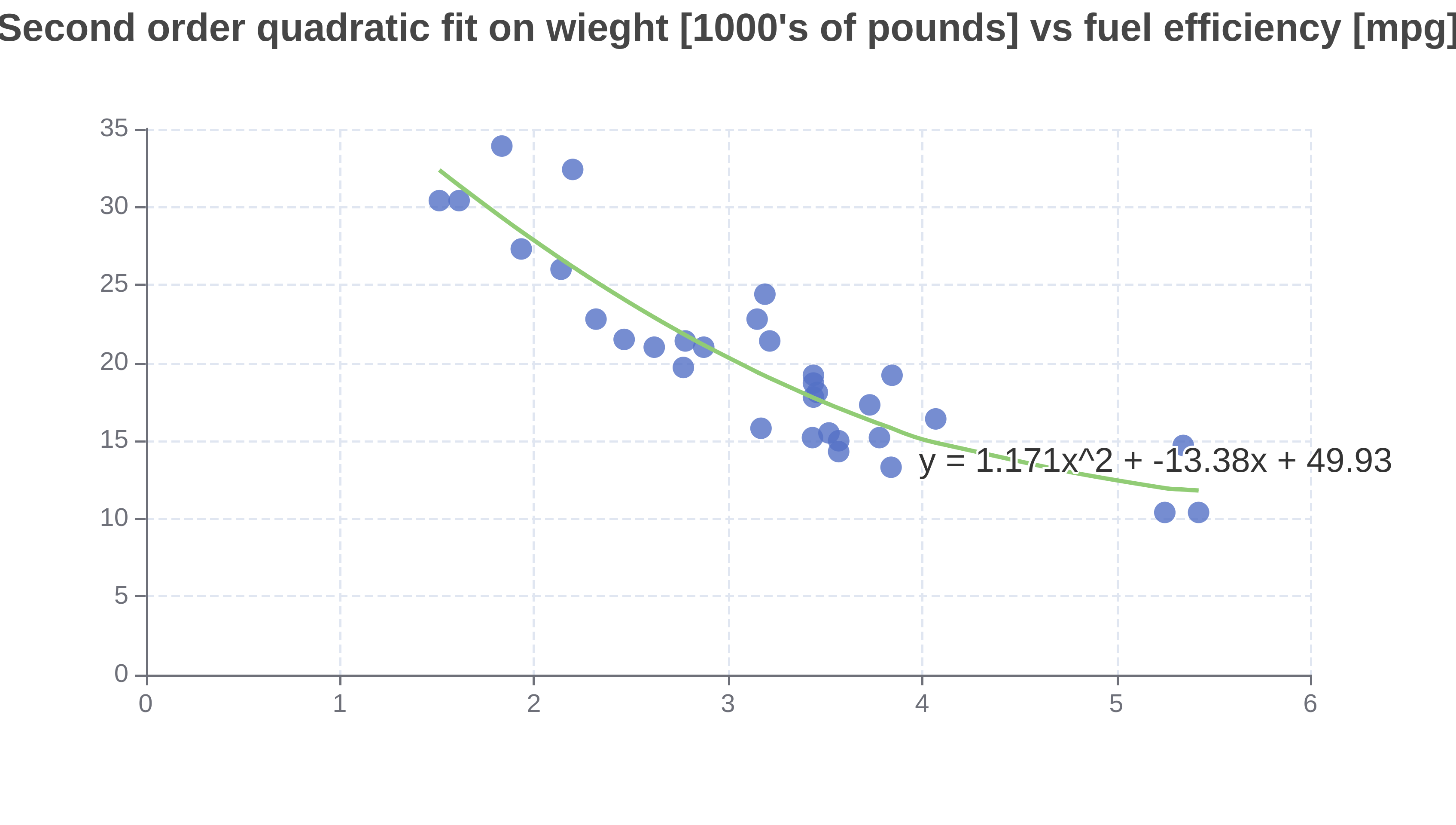The linear, quadratic, and polynomial regression calculators all use a linear model and least squares method to find a best fit line for the input data points. In this case, the linear model refers to the regression equations and not the shape of the best fit line.

The general form of a linear regression model is shown below:## How To Perform Exponential Regression

Click the dataset input at the top of the page. Or drag and drop your dataset into the input box.

2. ### Calculate exponential regression

The exponential regression calculator will calculate an exponential regression fit for you

## Exponential Regression Equation

The exponential regression calculator fits the following exponential model to the input data points.Where y is the predicted value for the response variable. a and b are coefficients fit by the exponential model.

The exponential fit is characterized by the parameters a and b. With a and b the regression line (best fit line) can be drawn.

If b is negative then the model has fit an exponential decay curve. If b is positive then the model has fit an exponential growth curve.

This exponential regression calculator can fit both exponential growth and exponential decay curves.